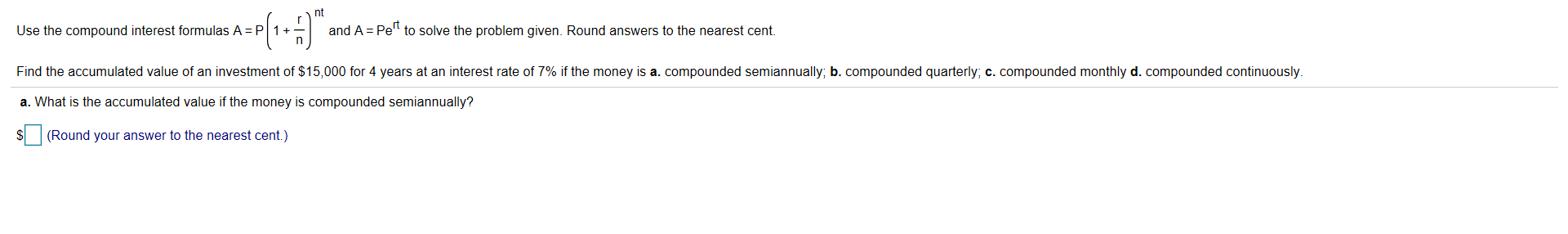# nt Use the compound interest formulas A P1 +_ and A Pe" to solve the problem given. Round answers to the nearest cent. Find the accumulated value of an investment of $15,000 for 4 years at an interest rate of 7% if the money is a" compounded semiannually, b. compounded quarterly, c. compounded monthly d. compounded continuously a. What is the accumulated value if the money is compounded semiannually? S(Round your answer to the nearest cent.) Questionhelp_outlineImage Transcriptionclosent Use the compound interest formulas A P1 +_ and A Pe" to solve the problem given. Round answers to the nearest cent. Find the accumulated value of an investment of$15,000 for 4 years at an interest rate of 7% if the money is a" compounded semiannually, b. compounded quarterly, c. compounded monthly d. compounded continuously a. What is the accumulated value if the money is compounded semiannually? S(Round your answer to the nearest cent.) fullscreen

## Expert Answer

### Want to see this answer and more?

Experts are waiting 24/7 to provide step-by-step solutions in as fast as 30 minutes!*

*Response times vary by subject and question complexity. Median response time is 34 minutes and may be longer for new subjects.
Tagged in© 2021 bartleby. All Rights Reserved.